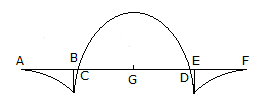# Civil Engineering - UPSC Civil Service Exam Questions

11.

Which is the best sewer material to resist hydrogen sulphide corrosion ?

 A. Glazed stone ware B. Glazed earthen ware C. R.C.C. D. Brick masonry

Explanation:

No answer description available for this question. Let us discuss.

12.

A steel bar of 2 m length is fixed at both ends at 20°C. The coefficient of thermal expansion is 11 x 10-6/°C and the modulus of the elasticity is 2 x 106 kg/cm2. If the temperature is changed to 18°C, then the bar will be experience a stress of

 A. 22 kg/cm2 (tensile) B. 22 kg/cm2 (compressive) C. 44 kg/cm2 (compressive) D. 44 kg/cm2 (tensile)

Explanation:

No answer description available for this question. Let us discuss.

13.

Which one of the following is not biodegradable organic matter ?

 A. Carbohydrates B. Fats C. Alcohols D. Petrol

Explanation:

No answer description available for this question. Let us discuss.

14.

A column base is subjected to moment. If the intensity of bearing pressure due to axial load is equal to stress due to the moment, then the bearing pressure between the base and the concrete is

 A. uniform compression throughout B. zero at one end and compression at the other end C. tension at one end and compression at the other end D. uniform tension throughout

Explanation:

No answer description available for this question. Let us discuss.

15.

The bending moment diagram for an overhanging beam is shown in the given figure :
The points of contraflexure would includeA. A and F B. B and E C. C and D D. A and D